2018-02-08 20:01:33 huayunhualuo 阅读数 3637
• ###### MATLAB图像处理

全面系统的学习MATLAB在图像处理中的应用

19870 人正在学习 去看看 魏伟

## 1 图像数据表示的层次

1. 图标图像(iconic images)：最底层的表示，有含有原始数据的图像组成，原始数据也就是像素亮度数据的整数矩阵。为了突出对后续处理重要 的图像的某些方面，需要进行预处理（滤波或边缘锐化）
2. 分割图像(segmented images)：为可能属于同一物体的区域。
3. 几何表示(geometric representation)：保存2D和3D形状知识。
4. 关系模型(relational models)：使我们能更有效地，并且在更高的抽象层次上的处理数据。

## 2 传统图像数据结构

### 2.1 矩阵

#### 特殊图像

• 二值图像(binary image)：仅有两个亮度级别的图像，用仅含有0和1的矩阵来表示
• 多光谱图像(multispectral image)：可以用多个矩阵来表示，每个矩阵含有一个频带的图像。
• 分层图像数据结构(bierarchical image data structures)：用不同的分辨率的矩阵来获得。

#### 共生矩阵(co-occurrence matrix)

​ 它是亮度为$z$$z$的像素$\left({i}_{1},{j}_{1}\right)$$(i_1,j_1)$和亮度为y的像素$\left({i}_{2},{j}_{2}\right)$$(i_2,j_2)$的具有空间关系的两个像素的概率估计。

​ 假设这个概率仅依赖于亮度$z$$z$的像素和亮度$y$$y$的像素之间的某个空间关系$r$$r$，那么关于关系$r$$r$的信息就记录在方形的共生矩阵C中，它的维数对应图像的亮度级别数。

1. ${C}_{r}\left(z,y\right)=0$$C_r(z,y) =0$,对于所有的$z,y\in \left[0,L\right]$$z,y\in[0,L]$,L是最大的亮度。
2. 对于图像中所有的像素$\left({i}_{1},{j}_{1}\right)$$(i_1,j_1)$ ，找到与像素$\left({i}_{1},{j}_{1}\right)$$(i_1,j_1)$有关系r的像素$\left({i}_{2}，{j}_{2}\right)$$(i_2，j_2)$,做${C}_{r}\left[f\left({i}_{1},{j}_{1}\right),f\left({i}_{2},{j}_{2}\right)\right]={C}_{r}\left[f\left({i}_{1},{j}_{1}\right),f\left({i}_{2},{j}_{2}\right)\right]+1$$C_r[f(i_1,j_1),f(i_2,j_2)] = C_r[f(i_1,j_1),f(i_2,j_2)]+1$.

#### 积分图像

​ 描述全局信息的矩阵表示方法。积分图像的构造方式是位置$\left(i,j\right)$$(i,j)$处的值$ii\left(i,j\right)$$ii(i,j)$是原图像的$\left(i,j\right)$$(i,j)$左上角所有像素的和：$ii\left(i,j\right)=\sum _{k\le i,l\le j}f\left(k,l\right)$$ii(i,j) = \sum\limits_{k\le i,l\le j}f(k,l)$

1. $s\left(i,j\right)$$s(i,j)$表示行方向的累加和，初始化$s\left(i,-1\right)=0$$s(i,-1) =0$

2. $ii\left(i,j\right)$$ii(i,j)$表示一个积分图像，初始化$ii\left(-1,i\right)=0$$ii(-1,i)=0$

3. 逐行扫描图像，递归计算每个像素$\left(i,j\right)$$(i,j)$行方向的累加和$s\left(i,j\right)$$s(i,j)$和积分图像$ii\left(i,j\right)$$ii(i,j)$的值

$s\left(i,j\right)=s\left(i,j-1\right)+f\left(i,j\right)$$s(i,j) = s(i,j-1)+f(i,j)$

$ii\left(i,j\right)=ii\left(i-1,j\right)+s\left(i,j\right)$$ii(i,j) =ii(i-1,j)+s(i,j)$

4. 扫描图像一遍，当到达图像右下角像素时，积分图像$ii$$ii$构造好了。

### 2.2 链

​ 链在计算机视觉中用于描述物体的边界。链的元素是一个基本符号，这种方法使得在计算机视觉任务中可以使用任何形式的理论。链适合组织成符号序列的数据，链中相邻的符号通常对应于图像中邻接的基元。

## 3 分层数据结构

### 3.1 金字塔

#### M型金字塔(M-pyramids)矩阵

M型金字塔：是一个图像序列$\left\{{M}_{L},{M}_{L-1},\cdots ,{M}_{0}\right\}$$\{M_L,M_{L-1},\cdots ,M_0\}$其中${M}_{L}$$M_L$是具有与原图像同样的分辨率和元素的图像${M}_{i-1}$$M_{i-1}$${M}_{i}$$M_i$降低一半分辨率得到的图像。通常我们只考虑维数是2的幂的方阵。

$M$$M$型金字塔存储所有图像矩阵需要的像素个数为${N}^{2}\left(1+\frac{1}{4}+\frac{1}{16}+\cdots \right)=1.33{N}^{2}$$N^2(1+\frac{1}{4}+\frac{1}{16}+\cdots) =1.33N^2$

#### T型金字塔(T-pyramids)树形

T型金字塔：树状结构。设${2}^{l}$$2^l$是原图像的大小（最高分辨率）。

1. 一个结点集合$P=\left\{P=\left(k,i,j\right)\right\}$$P=\{P=(k,i,j)\}$使得级别$k\in \left[0,L\right]$$k\in [0,L]$$i,j\in \left[0,{2}^{k}-1\right]$$i,j\in[0,2^k-1]$
2. 一个映射$F$$F$，定义在金字塔的结点${P}_{k-1},{P}_{k}$$P_{k-1},P_k$之间$F(k,i,j) = (k-1,i\ div \ 2,j\ div\ 2)$$div$$div$表示整除
3. 一个函数$V$$V$，将金字塔的结点$P$$P$映射到$Z$$Z$，其中$Z$$Z$是对应于亮度级别数的所有数的子集合

特点：

4. 对于给定的$k,T$$k,T$型金字塔的结点对应于M型金字塔的一些图像点，结点$P=\left\{\left(k,i,j\right)\right\}$$P=\{(k,i,j)\}$集合的每个元素对应于M型金字塔的一个矩阵，称$k$$k$为金字塔的层数。

5. 对于给定的$k$$k$ ，图像$P=\left\{\left(k,i,j\right)\right\}$$P =\{(k,i,j)\}$构成金字塔的第$k$$k$层的一个图像。
6. $F$$F$是所谓的父亲映射，在T型金字塔中，除了根$\left(0,0,0\right)$$(0,0,0)$之外的所有的结点${P}_{k}$$P_k$都有定义。
7. 除了叶子结点外，T型金字塔的每个结点都有4个子结点，叶子结点是第L层的结点，对应于图像的单个像素。

### 3.2 四叉树

​ 对T型金字塔的改进，除叶子结点外每个结点有4个子结点(西北NW，东北NE，西南SW，东南SE)，在每个层次图像被分解为4个象限。

2018-04-21 15:45:00 learning_tortosie 阅读数 10012
• ###### MATLAB图像处理

全面系统的学习MATLAB在图像处理中的应用

19870 人正在学习 去看看 魏伟

# 实验目的

1．理解并掌握形态学图像处理中的开操作和闭操作
2．熟悉并掌握MATLAB软件的使用

# 相关知识

## 1.定义

$A○B=\left(A\ominus B\right)\oplus B$

$A•B=\left(A\oplus B\right)\ominus B$

## 2.几何解释

（1）开操作的何解释
A○B的边界由B中的点建立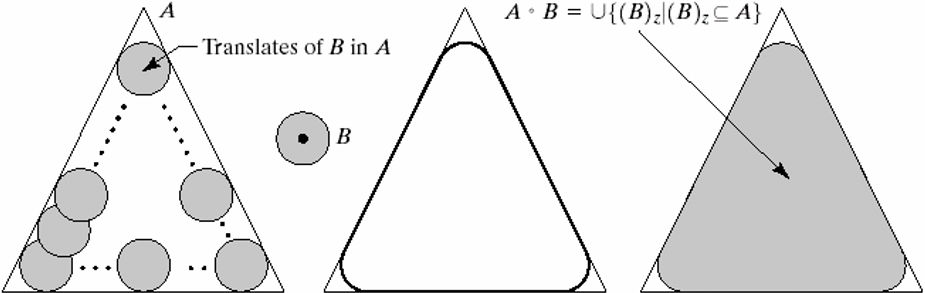（2）闭操作的几何解释
A•B的边界由B中的点建立
B在A的边界外侧滚动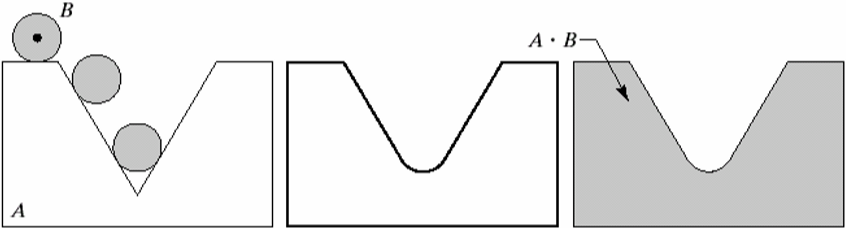## 3.相关函数说明

（1）strel

SE = STREL('arbitrary',NHOOD,HEIGHT) 创建一个指定领域的非平面结构化元素。HEIGHT是一个矩阵，大小和NHOOD相同，他指定了NHOOD中任何非零元素的高度值。
SE = STREL('ball',R,H,N) 创建一个空间椭球状的结构元素，其X-Y平面半径为R，高度为H。R必须为非负整数，H是一个实数。N必须为一个非负偶数。当N>0时此球形结构元素由一系列空间线段结构元素来近似。
SE = STREL('diamond',R) 创建一个指定大小R平面钻石形状的结构化元素。R是从结构化元素原点到其点的距离，必须为非负整数。
SE = STREL('disk',R,N) 创建一个指定半径R的平面圆盘形的结构元素。这里R必须是非负整数. N须是0, 4, 6, 8.当N大于0时,圆盘形结构元素由一组N(或N+2)个周期线结构元素来近似。当N等于0时,不使用近似，即结构元素的所有像素是由到中心像素距离小于等于R的像素组成。N可以被忽略,此时缺省值是4。注: 形态学操作在N>0情况下要快于N=0的情形。

（3）imdilate

# 实验内容

a 图是受噪声污染的指纹的二值图像，噪声为黑色背景上的亮元素和亮指纹部分的暗元素
b图是使用的结构元
c图是使用结构元素对图a腐蚀的结果：背景噪声消除了，指纹中的噪声尺寸增加
d图是使用结构元素对图c膨胀的结果：包含于指纹中的噪声分量的尺寸被减小或被完全消除，带来的问题是：在指纹纹路间产生了新的间断
e图是对图d膨胀的结果，图d的大部分间断被恢复，但指纹的线路变粗了
f图是对图e腐蚀的结果，即对图d中开操作的闭操作。最后结果消除了噪声斑点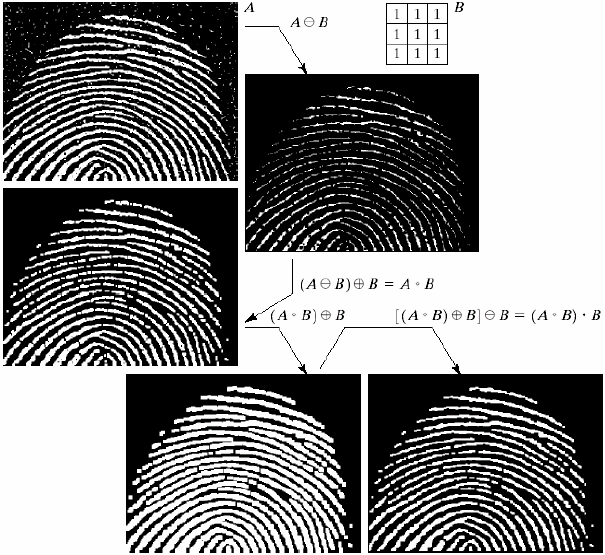# 实验结果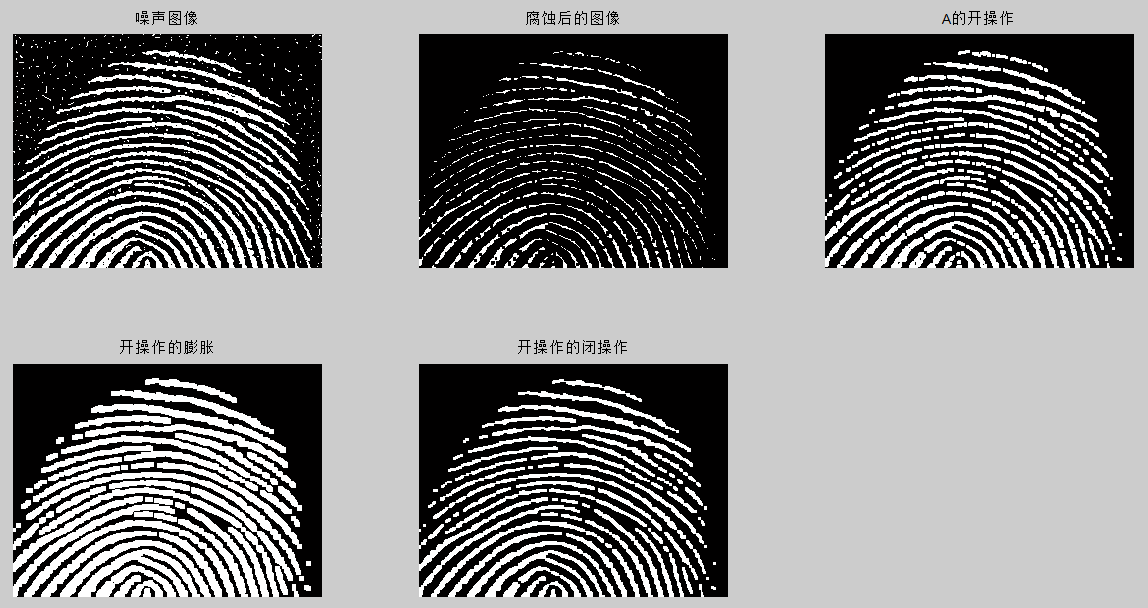# 附录

## matlab程序

A=imread('Fig0911(a)(noisy_fingerprint).tif'); %注意图片的路径要设置正确
subplot(2,3,1);
imshow(A)
title('噪声图像')
se=strel('square',3);
A2=imerode(A,se);
subplot(2,3,2);
imshow(A2)
title('腐蚀后的图像')
A3=imopen(A,se);
subplot(2,3,3);
imshow(A3)
title('A的开操作')
A4=imdilate(A3,se);
subplot(2,3,4);
imshow(A4)
title('开操作的膨胀')
A5=imclose(A3,se);
subplot(2,3,5);
imshow(A5)
title('开操作的闭操作')

2019-09-01 19:51:41 webzhuce 阅读数 385
• ###### MATLAB图像处理

全面系统的学习MATLAB在图像处理中的应用

19870 人正在学习 去看看 魏伟

# 基本概念

“骨架”是指一幅图像的骨骼部分，它描述物体的几何形状和拓扑结构，是重要的图像描绘子之一。计算骨架的过程一般称为“细化”或“骨架化”，在包括文字识别、工业零件形状识别以及印刷电路板自动检测在内的很多应用中，细化过程都发挥这关键作用。通常，对我们感兴趣的目标物体进行细化有助于突出目标的形状特点和拓扑结构并且减少冗余的信息。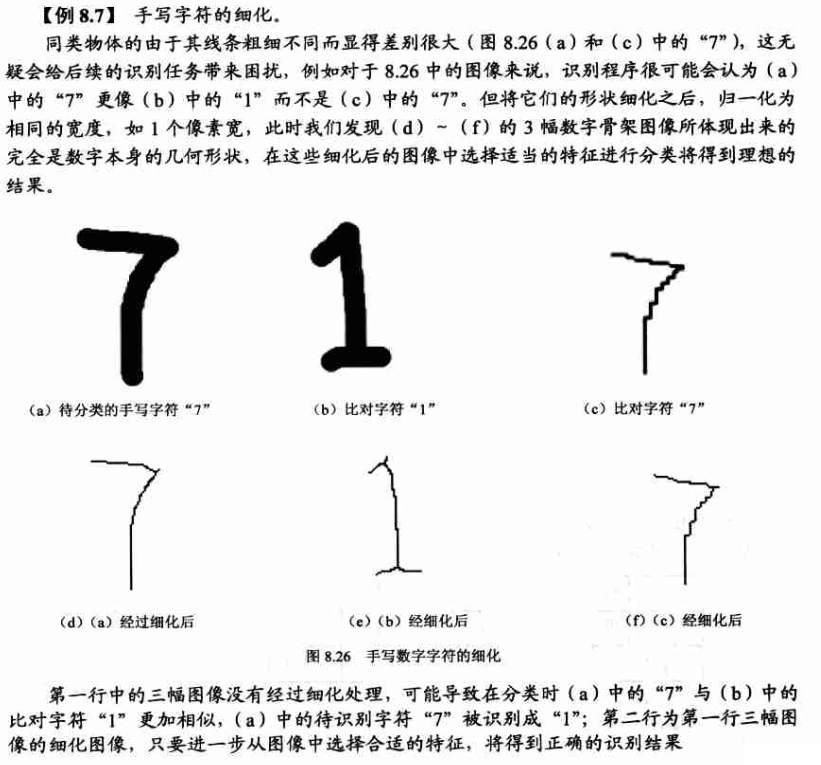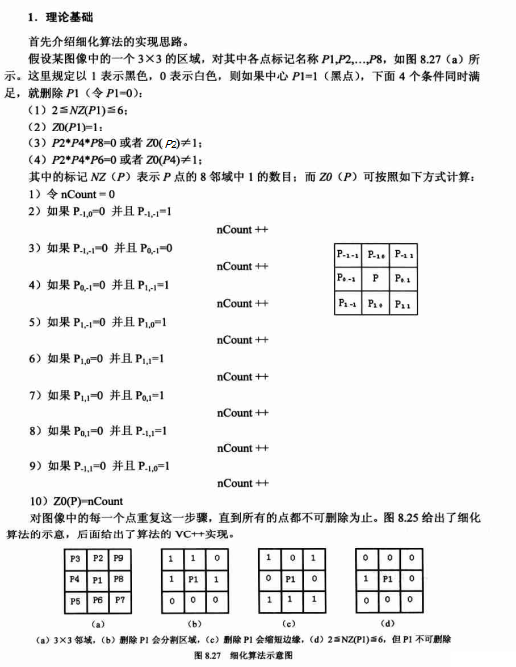# 示例演示

实现基于形态学的细化算法。与上面算法介绍不同，将白色作为前景，黑色作为背景。完整工程代码。

/*
*only process binary image
* white is foreground
*/
void Thining(const cv::Mat &src, cv::Mat &dst)
{

//four conditions
bool condition1 = false, condition2 = false, condition3 = false, condition4 = false;

//n*n neighbours
constexpr int N = 5;
uchar neighbour[N][N];
const int kOffset = N / 2;
src.copyTo(dst);

bool modified = true;
while(modified)
{
modified = false;
Mat img;
dst.copyTo(img);

for(int i = kOffset; i < (dst.rows - kOffset); i++)
{
for(int j = kOffset; j < (dst.cols - kOffset); j++)
{
if(dst.at<uchar>(i, j) == 0)
continue;

condition1 = false;
condition2 = false;
condition3 = false;
condition4 = false;

//get N*N neighbours of current point
//white : 0, black : 1
for(int m = 0; m < N; m++)
{
for(int n = 0; n < N; n++)
{
neighbour[m][n] = dst.at<uchar>(i + n - kOffset, j + m - kOffset) == 255 ? 1 : 0;
}
}

//2 <= NZ(P1) <=6
int count = neighbour + neighbour + neighbour +
neighbour + neighbour +
neighbour + neighbour + neighbour;
if(count >= 2 && count <=6)
condition1 = true;

//Z0(P1) == 1
count = 0;
if(neighbour == 0 && neighbour == 1)
count++;
if(neighbour == 0 && neighbour == 1)
count++;
if(neighbour == 0 && neighbour == 1)
count++;
if(neighbour == 0 && neighbour == 1)
count++;
if(neighbour == 0 && neighbour == 1)
count++;
if(neighbour == 0 && neighbour == 1)
count++;
if(neighbour == 0 && neighbour == 1)
count++;
if(neighbour == 0 && neighbour == 1)
count++;
if(count == 1)
condition2 = true;

//P2*P4*P8 = 0 or Z0(P2) != 1
if(neighbour * neighbour * neighbour == 0)
condition3 = true;
else
{
count = 0;
if(neighbour == 0 && neighbour == 1)
count++;
if(neighbour == 0 && neighbour == 1)
count++;
if(neighbour == 0 && neighbour == 1)
count++;
if(neighbour == 0 && neighbour == 1)
count++;
if(neighbour == 0 && neighbour == 1)
count++;
if(neighbour == 0 && neighbour == 1)
count++;
if(neighbour == 0 && neighbour == 1)
count++;
if(neighbour == 0 && neighbour == 1)
count++;
if(count != 1)
condition3 = true;
}

//P2*P4*P6 = 0 or Z0(P4) != 1
if(neighbour * neighbour * neighbour == 0)
condition4 = true;
else
{
count = 0;
if(neighbour == 0 && neighbour == 1)
count++;
if(neighbour == 0 && neighbour == 1)
count++;
if(neighbour == 0 && neighbour == 1)
count++;
if(neighbour == 0 && neighbour == 1)
count++;
if(neighbour == 0 && neighbour == 1)
count++;
if(neighbour == 0 && neighbour == 1)
count++;
if(neighbour == 0 && neighbour == 1)
count++;
if(neighbour == 0 && neighbour == 1)
count++;
if(count != 1)
condition4 = true;
}

if(condition1 && condition2 && condition3 && condition4)
{
img.at<uchar>(i, j) = 0;
modified = true;
}
else
img.at<uchar>(i, j) = 255;

} // for columns
} // for rows
img.copyTo(dst);
} //  for while
}


## 运行结果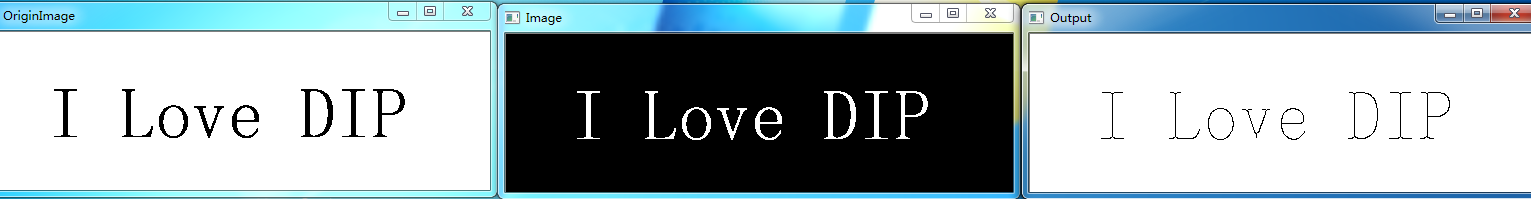2016-07-06 20:50:26 zhougynui 阅读数 2601
• ###### MATLAB图像处理

全面系统的学习MATLAB在图像处理中的应用

19870 人正在学习 去看看 魏伟

定义：对集合A做腐蚀操作，然后对结果再进行膨胀操作。

公式：功能：平滑屋里的轮廓、断开较窄的狭颈并消除细的突出物。

原理说明：把结构元素B视为一个（扁平）的“转球”，B对A的开操作的边界由B中的点建立：当B在A的边界内侧滚动时，B所能到达的A的边界的最远点。即为右侧图中黑色线部分。定义：结构元素B对集合A的闭操作就是简单的用B对A膨胀，紧接着用B对结果进行腐蚀。

公式：功能：平滑轮廓的一部分，弥合较窄的间断和细长的沟壑，消除小的空洞，填充轮廓线中的断裂。

原理说明：把结构元素B视为一个（扁平）的“转球”，B对A的开操作的边界由B中的点建立：当B在A的边界外侧滚动时，B所能到达的A的边界的最远点。即为右侧图中黑色线部分。2019-04-11 17:23:14 qq_35789421 阅读数 185
• ###### MATLAB图像处理

全面系统的学习MATLAB在图像处理中的应用

19870 人正在学习 去看看 魏伟

1.形态学梯度

2.顶帽和黑帽运算

void morphologyEx(InputArray src,OutputArrat dst,int op,InputArray kernel,Point anchor = Point(-1,-1),int iterations = 1 ,int morph-ologyDefaultBorderValue())